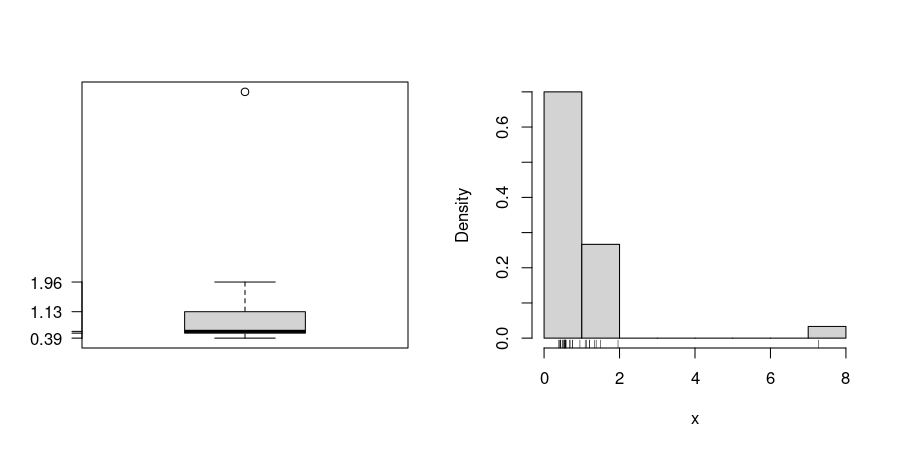## Exam 1

1. #### Question

Consider the following table:

Name Length Weight
Fritz 187 85
Wilhelm 161 66
Dieter 163 66
Detlef 195 98

What is the average of the variable “Length”?

#### Solution

The average “Length” is 176.5:

$\bar x = \frac{187 + 161 + 163 + 195}{4} = 176.5.$

2. #### Question

A machine fills milk into $$250$$ml packages. It is suspected that the machine is not working correctly and that the amount of milk filled differs from the setpoint $$\mu_0 = 250$$. A sample of $$157$$ packages filled by the machine are collected. The sample mean $$\bar{y}$$ is equal to $$240.8$$ and the sample variance $$s^2_{n-1}$$ is equal to $$137.67$$.

Test the hypothesis that the amount filled corresponds on average to the setpoint. What is the value of the t-test statistic?

1. $$25.240$$
2. $$-9.825$$
3. $$-9.495$$
4. $$5.415$$
5. $$28.504$$

#### Solution

The t-test statistic is calculated by: \begin{aligned} t & = & \frac{\bar y - \mu_0}{\sqrt{\frac{s^2_{n-1}}{n}}} = \frac{240.8 - 250}{\sqrt{\frac{137.67}{157}}} = -9.825. \end{aligned} The t-test statistic is thus equal to $$-9.825$$.

1. False
2. True
3. False
4. False
5. False

3. #### Question

In a small city the satisfaction with the local public transportation is evaluated. One question of interest is whether inhabitants of the city are more satisfied with public transportation compared to those living in the suburbs.

A survey with 250 respondents gave the following contingency table:

           Location
Evaluation  City Suburbs
Very good   18      22
Good        36      23


The following table of percentages was constructed:

           Location
Evaluation  City    Suburbs
Very good    18.0    14.7
Good         36.0    15.3


Which of the following statements are correct?

1. The percentage table provides the satisfaction distribution for each location type.
2. The percentage table provides row percentages.
3. The value in row 1 and column 2 in the percentage table indicates: 14.7 percent of those living in the suburbs evaluated the public transportation as very good.
4. The value in row 2 and column 2 in the percentage table indicates: 15.3 percentage of those, who evaluated the public transportation as good live in the suburbs.
5. The percentage table can be easily constructed from the original contingency table: percentages are calculated for each column.

#### Solution

In the percentage table, the column sums are about 100 (except for possible rounding errors). Hence, the table provides column percentages, i.e., conditional relative frequencies for satisfaction level given location type.

1. True. The column sums are equal to 100 (except for possible rounding errors).
2. False. The row sums are not equal to 100.
3. True. This is the correct interpretation for column percentages.
4. False. This is an interpretation for row percentaes, but the table provides column percentages.
5. True. This calculation yields column percentages.

4. #### Question

Consider the following regression results:


Call:
lm(formula = log(y) ~ x, data = d)

Residuals:
Min       1Q   Median       3Q      Max
-1.45662 -0.31421 -0.04617  0.26287  1.27416

Coefficients:
Estimate Std. Error t value Pr(>|t|)
(Intercept)  0.03675    0.06787   0.541     0.59
x           -0.74279    0.05701 -13.030   <2e-16 ***
---
Signif. codes:  0 '***' 0.001 '**' 0.01 '*' 0.05 '.' 0.1 ' ' 1

Residual standard error: 0.4987 on 52 degrees of freedom
Multiple R-squared:  0.7655,    Adjusted R-squared:  0.761
F-statistic: 169.8 on 1 and 52 DF,  p-value: < 2.2e-16


Describe how the response y depends on the regressor x.

#### Solution

The presented results describe a semi-logarithmic regression.

The mean of the response y decreases with increasing x.

If x increases by 1 unit then a change of y by about -52.42 percent can be expected.

Also, the effect of x is significant at the 5 percent level.

5. #### Question

For the 30 observations of the variable x in the data file boxhist.csv draw a histogram, a boxplot and a stripchart. Based on the graphics, answer the following questions or check the correct statements, respectively. (Comment: The tolerance for numeric answers is $$\pm0.3$$, the true/false statements are either about correct or clearly wrong.)

1. The distribution is unimodal. / The distribution is not unimodal.
2. The distribution is symmetric. / The distribution is right-skewed. / The distribution is left-skewed.
3. The boxplot shows outliers. / The boxplot shows no outliers.
4. A quarter of the observations is smaller than which value?
5. A quarter of the observations is greater than which value?
6. Half of the observations are greater than which value?

#### Solution1. True / False
2. False / True / False
3. True / False
4. 0.53
5. 1.13
6. 0.58